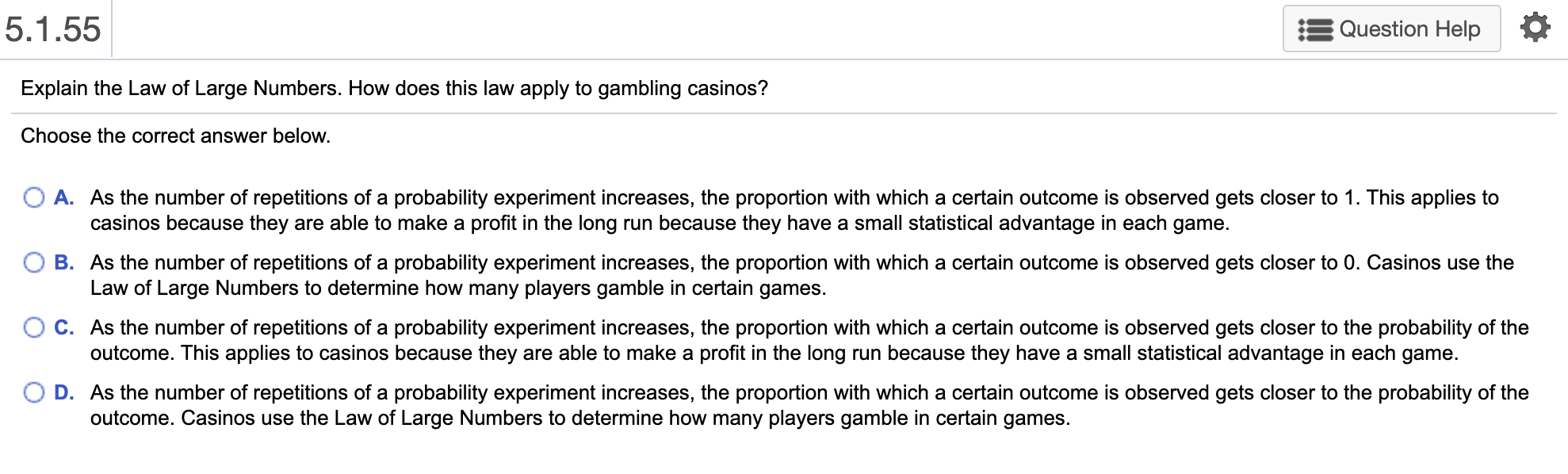# Explain the law of large numbers. how does this law apply to gambling casinos?5.1.55 Question Help Explain the Law of Large Numbers. How does this law apply to gambling casinos? Choose the correct answer below. O A. As the number of repetitions of a probability experiment increases, the proportion with which a certain outcome is observed gets closer to 1. This applies to casinos because they are able to make a profit in the long run because they have a small statistical advantage in each game. B. As the number of repetitions of a probability experiment increases, the proportion with which a certain outcome is observed gets closer to 0. Casinos use the Law of Large Numbers to determine how many players gamble in certain games. O C. As the number of repetitions of a probability experiment increases, the proportion with which a certain outcome is observed gets closer to the probability of the outcome. This applies to casinos because they are able to make a profit in the long run because they have a small statistical advantage in each game. D. As the number of repetitions of a probability experiment increases, the proportion with which a certain outcome is observed gets closer to the probability of the outcome. Casinos use the Law of Large Numbers to determine how many players gamble in certain games.

Solution
The answer is, ” C. As the number of repetitions of a
probability experiment increases, the proportion with which a
certain outcome is observed gets closer to the probability of
the
outcome. This applies to casinos because they are able to make a
profit in the long run because they have a small statistical